# Acoustics and Vibration Animations

Daniel A. Russell, Graduate Program in Acoustics, The Pennsylvania State UniversityThis work by Dan Russell is licensed under a Creative Commons Attribution-NonCommercial-NoDerivatives 4.0 International License.

# Standing Sound Waves (Longitudinal Standing Waves)

## The problem: using static graphs to depict standing waves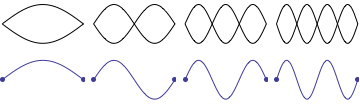While teaching undergraduate physics at Kettering University for 16 years, I was often frustrated with the depiction of standing sound waves in pipes as it was presented in most elementary physics textbooks. Even textbooks specializing in wave phenomena were often not much better. Students are generally introduced to the concept of standing waves through a discussion of transverse standing waves on a string. Static images of standing waves on a fixed-fixed string are more readily understood intuitively because the static "graphs" showing standing wave patterns correspond directly to the transverse displacment of the string, as depicted in the animation shown. As the string moves up and down the displacement fits into the envelope of the static graphs representing the standing wave patterns.

However, sound waves are longitudinal waves and the particle motion associated with a standing sound wave in a pipe is directed along the length of the pipe (back and forth along the pipe axis, or left and right horizontally for the images shown at right). It is a bit more difficult to imagine horizontal motion depicted by graphs that appear to show vertical displacement, and this is the problem I have with most textbook treatments of standing sound waves. For example, Figure 6-6 shown at the right is from a specialized textbook that I used for several years while teaching a junior level physics of waves course to physics majors. The figure caption states that the figure shows "standing waves in a pipe" but does not indicate what quantity (displacement or pressure?) is being represented by this "standing wave profile." The caption also mentions the "amplitude of the standing wave" but again does not define what that amplitude represents. The answer is that he curves in the figure represent the extremes of the horizontal particle displacement amplitude of the air molecules as the standing wave oscillates through a complete cycle, but such an interpretation is not readily apparent from such a graph, and certainly not apparent from this figure caption.

Other textbooks (like the Prentice Hall physics textbook from which the second image at right is borrowed) try to do better by showing two companion sets of graphs, one showing the displacement of air an the other for pressure associated with the sound wave. However, the displacement plots still don't clearly indicate that the displacement amplitude is for a longitudinal horizontal (left-to-right) motion of the air particles.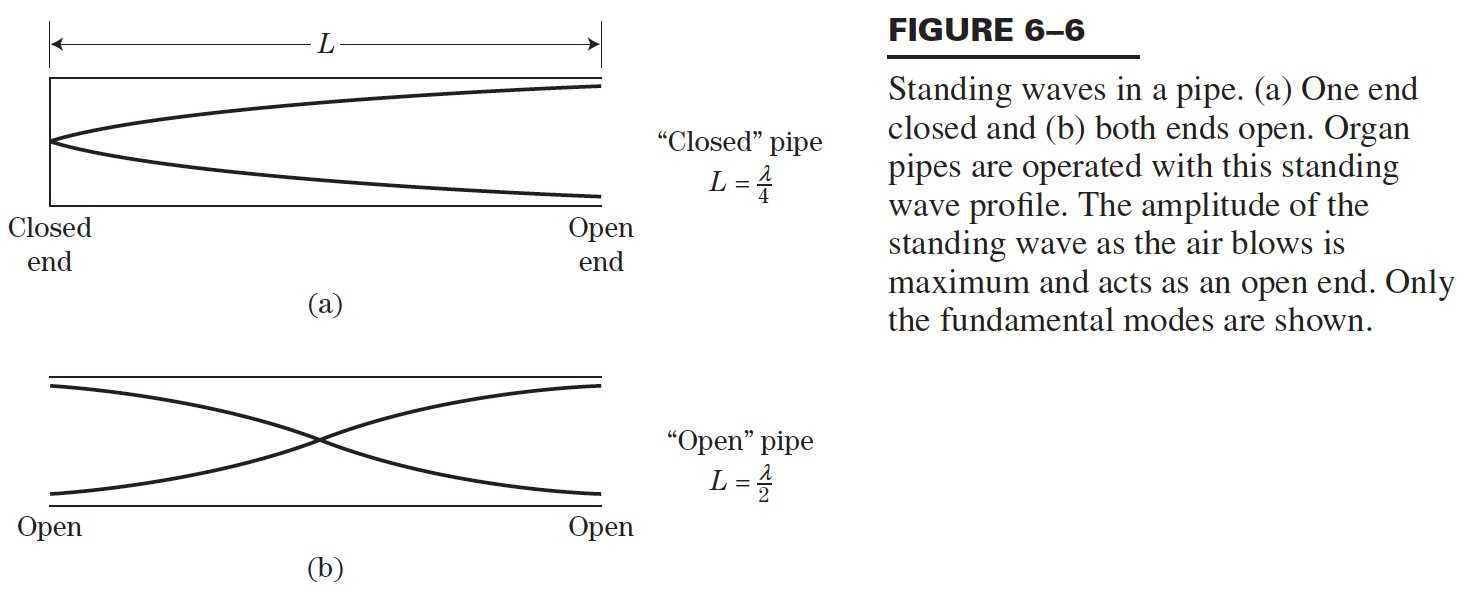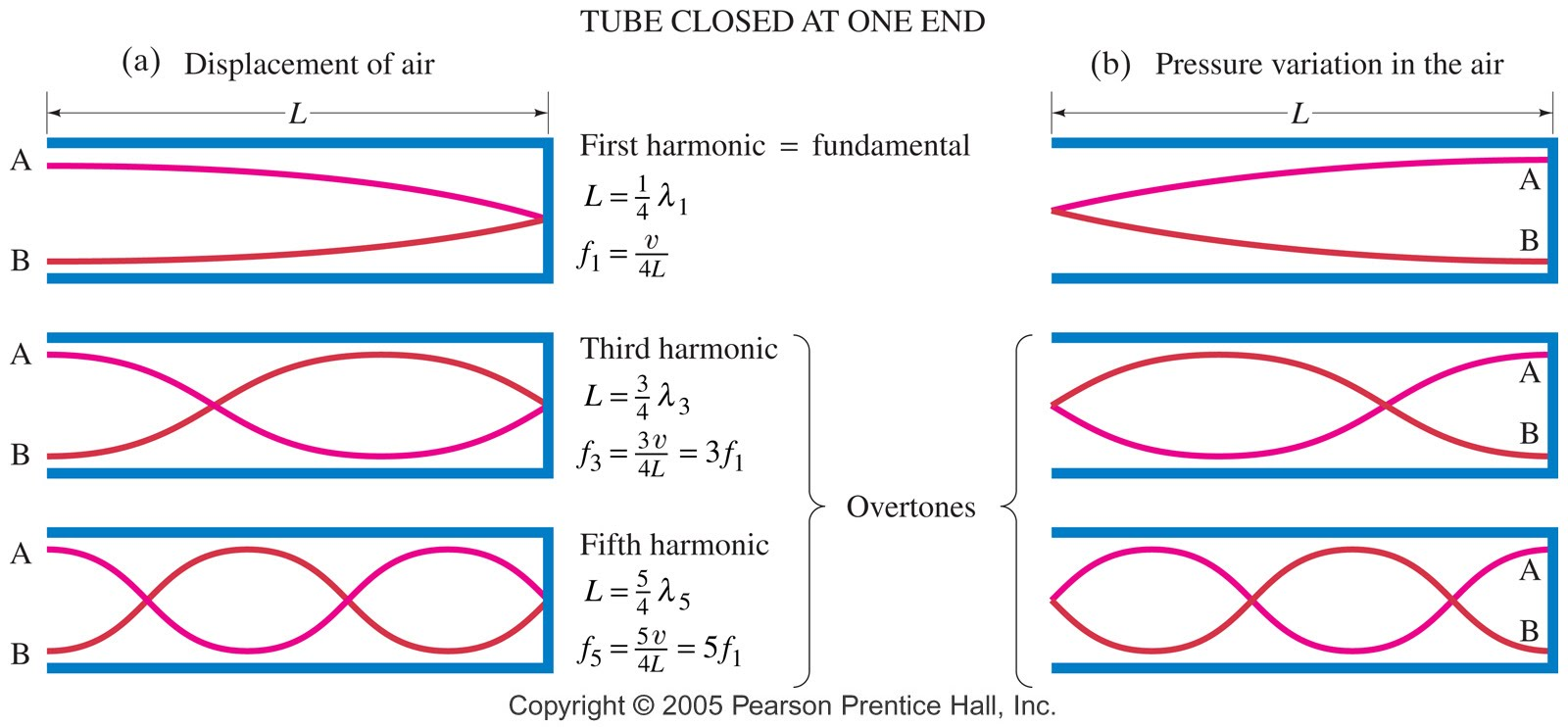There are a couple of websites that try to correctly explain what the pictures mean (distinguishing between pressure and longitudinal particle motion):

I created the animation below and its accompanying description in an attempt to better explain the behavior of a standing sound wave in a pipe.

## The solution: an animation to visualize particle motion and pressure for longitudinal sound waves.

The particular example of a standing wave that I want to illustrate is a standing sound wave in a pipe that is forced (by a moving piston or loudspeaker) at the left end and closed at the right end. This is the situation depicted by the figure from the Prentice Hall textbook, shown above and animated at right. The piston at the left end of the tube oscillates back and forth at a frequency equal to the ninth harmonic of the fundamental frequency of this "quarter-wavelength resonator." I'll describe each of the three plots that comprise the animation in turn.

### Top animation: actual standing wave particle motion.

The particles immediately to the right in front of the piston move with the piston as it oscillates back and forth. Elsewhere in the pipe, the particles oscillate back and forth, right and left, though they are not all moving in the same direction at the same time; some are moving to the right while others are moving to the left. And, you should notice several locations (four, to be exact) where the particles do not move at all. I've placed three red particles in the pipe to illustrate the extremes of motion. One of the red particles does not move at all -- it is located at a displacement node, a location where the amplitude of the displacement always zero. Actually, the node is a line or plane that extends across the entire cross-section of the pipe. Notice that the particles to the right and left of this stationary node alternately move inward toward the node or outward away from the node. As the particles move toward the node, they become closer together and the local particle density at the node location increases (this would represent a compression). As the particles move outward away from the node the local particle density at the node location decreases (a rarefaction). To the right of this stationary red particle is another red particle that moves with maximum displacement; this particle is located at a displacement antinode. Notice that the local particle density near an antinode does not change as the particles move back and forth. The closed end of the pipe acts as a displacement node; the particles cannot move beyond the rigid end, so the displacement is zero at the closed end. The piston face acts as a displacement antinode; the particles move with maximum displacement amplitude.

(animation may take a few seconds to load . . . please be patient)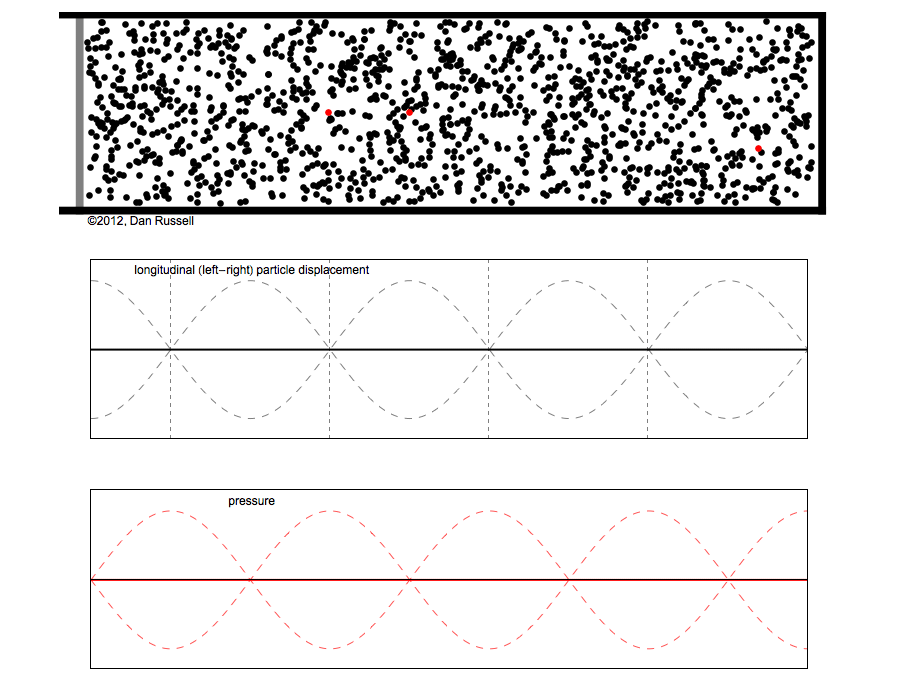### Middle animation: graph representing longitudinal particle displacement.

The middle animation shows a graph representing the horizontal displacement of the air particles in the standing wave. When the graph is a horizontal line at zero all of the particles are at their equilibrium positions. Regions where the graph becomes positive represent regions where the particles are displaced from their equilibrium locations toward the right, in the positive x-direction. Regions where the graph becomes negative represent regions where the particles are displaced from their equilibrium positions toward the left, in the negative x-direction. Locations where the particle displacement graph is always zero correspond to the to the displacement nodes.

### Bottom animation: graph representing pressure variation.

The bottom animation shows a graph representing the pressure variation associated with this standing sound wave. When the local density of the particles increases above the ambient value, the pressure variation is positive; this occurs when the particles are moving inward toward a displacement node location. When the local density of the particles decreases below the ambient value, the pressure variation is negative; this occurs when the particles move outward away from a displacement node location. If you compare the three animations, you'll notice that the pressure nodes (locations where the pressure is always zero) coincide with the displacement antinodes, there the local particle density does not change as the particles move back and forth together.

### Additional Animations for the First Four Standing Wave Patterns.

For a pipe closed at one end and driven at the open end, the natural (resonance) frequencies are odd integer multiples of the fundamental. The standing wave pattern shown above is actually the 5th mode, or the ninth harmonic, with a frequency 9 times the fundamental. Below are the animations for the other four standing wave patterns that precede the pattern shown above. The speed of the animations below is approximately representative of the actual frequency relative to the fundamental; the frequency of the 3rd harmonic would be 3 times the frequency of the fundamental.
Below left is the fundamental mode (the quarter wavelength mode). On the right is the second mode (third harmonic).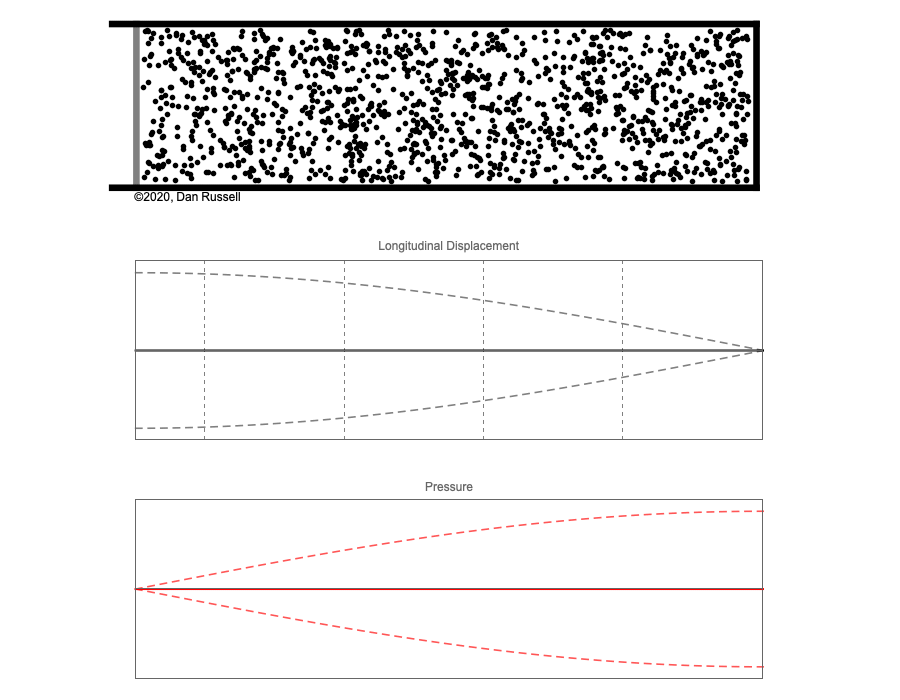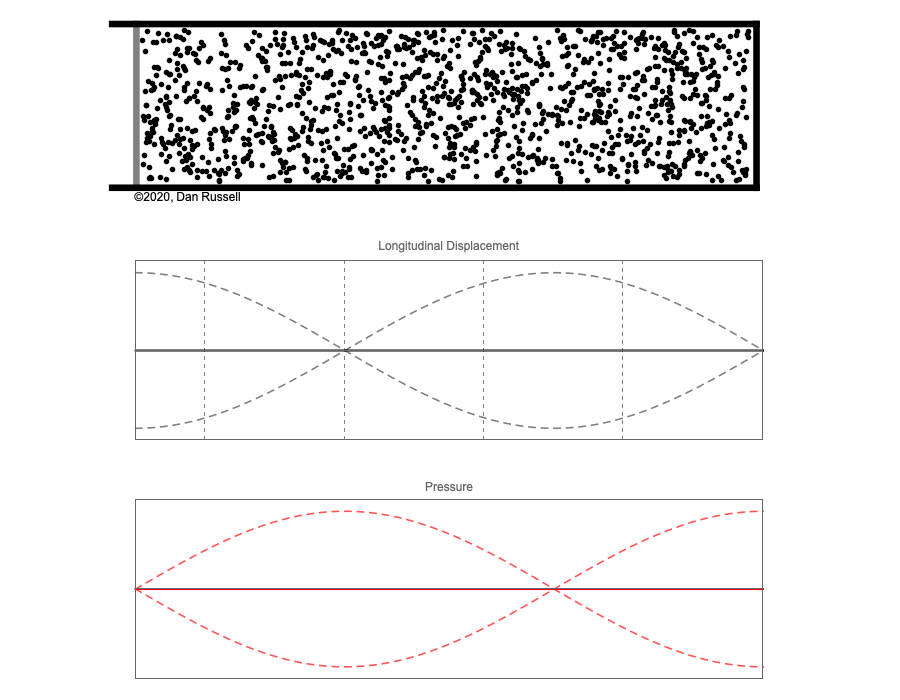Below left is the third mode (fifth harmonic) and below right is the fourth mode (7th harmonic).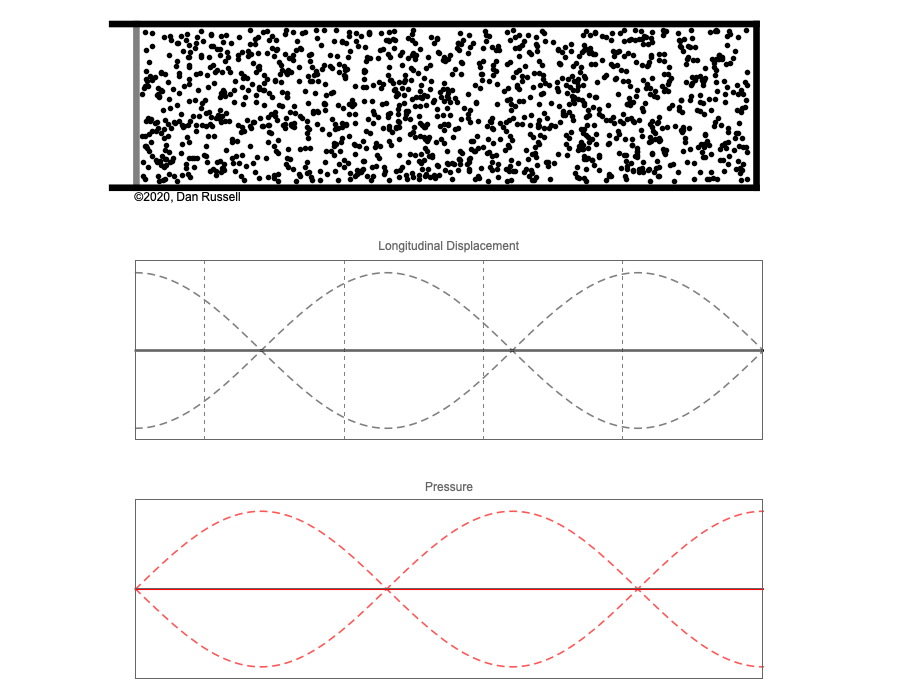### References

• Akira Hirose and Karl E. Lonngren, Fundamentals of Wave Phenomena, 2nd Ed., (SciTech Publishing, 2010). ISBN: 978-1-891121-92-0.